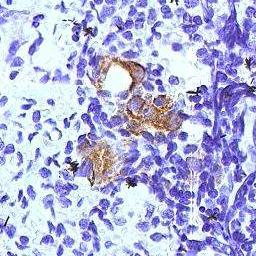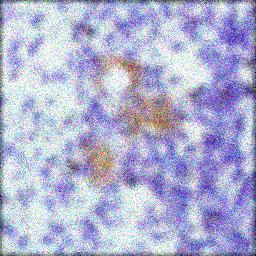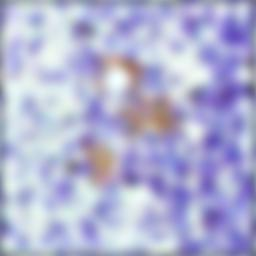Image Processing Toolbox User's GuideDeblurring with a Regularized Filter

Use the `deconvreg` function to deblur an image using a regularized filter. A regularized filter can be used effectively when limited information is known about the additive noise.

To illustrate, this example simulates a blurred image by convolving a Gaussian filter PSF with an image (using `imfilter`). Additive noise in the image is simulated by adding Gaussian noise of variance `V` to the blurred image (using `imnoise`):

1. Read an image into the MATLAB workspace. The example uses cropping to reduce the size of the image to be deblurred. This is not a required step in deblurring operations.
• ```I = imread('tissue.png');
I = I(125+[1:256],1:256,:);
figure; imshow(I); title('Original Image');Image Courtesy Alan W. Partin
```
2. Create the PSF.
• ```PSF = fspecial('gaussian',11,5);
```
3. Create a simulated blur in the image and add noise.
• ```Blurred = imfilter(I,PSF,'conv');

V = .02;
BlurredNoisy = imnoise(Blurred,'gaussian',0,V);
figure;imshow(BlurredNoisy);title('Blurred and Noisy Image');```
4. Use `deconvreg` to deblur the image, specifying the PSF used to create the blur and the noise power, `NP`.
• ```NP = V*prod(size(I));
[reg1 LAGRA] = deconvreg(BlurredNoisy,PSF,NP);
figure,imshow(reg1),title('Restored Image');```

Refining the Result

You can affect the deconvolution results by providing values for the optional arguments supported by the `deconvreg` function. Using these arguments you can specify the noise power value, the range over which `deconvreg` should iterate as it converges on the optimal solution, and the regularization operator to constrain the deconvolution. To see the impact of these optional arguments, view the Image Processing Toolbox deblurring demos.Deblurring with the Wiener Filter Deblurring with the Lucy-Richardson Algorithm